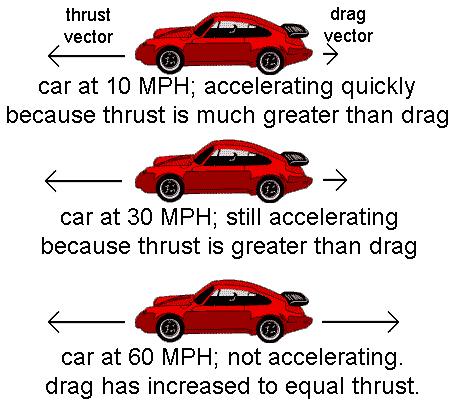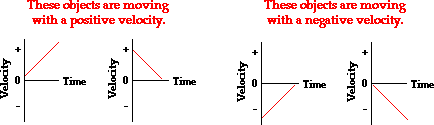# Velocity and Acceleration

• Astrophysics• Electricity• Electromagnetism• Energy• Fields• Force• Mechanics• Momentum• Nuclear Physics• Quantities & Units• States of Matter• Waves• Key ExperimentsVelocity is the rate at which an object moves in a particular direction. It's different from speed, which only tells us how fast something is going. Acceleration, on the other hand, is the rate at which an object changes its velocity. So, even if we know the velocity of an object, we still need to know its acceleration to understand how it's moving.

To calculate velocity or acceleration, we use specific equations. When we graph these concepts with respect to time, we can see how they change over time. Let's take a look at a few examples to help us better understand velocity and acceleration.

So, in summary, velocity and acceleration are both important concepts in understanding how objects move. By learning about their definitions, equations, and graphs, we can gain a deeper understanding of these concepts. Happy learning!

## Velocity and acceleration formula

The velocity of an object is its speed in a given direction. Velocity is a vector quantity.

Velocity is a measure of how fast an object is moving in a particular direction. We can calculate velocity using an equation that takes into account the change in displacement (distance in a particular direction) of the object over a certain period of time. Velocity is usually measured in meters per second (m/s).

The difference between speed and velocity is that speed only tells us how fast something is going, while velocity tells us how fast it's going in a certain direction. Speed is a scalar quantity, meaning it only has a magnitude, while velocity is a vector quantity, meaning it has both a magnitude and a direction. For example, if a car is traveling at 60 miles per hour, that's only its speed. But if the car is traveling at 60 miles per hour due north, that's its velocity because it has both a speed and a direction.

Acceleration is the rate at which an object's velocity changes over time. For example, when a car speeds up or slows down, it's experiencing acceleration. Acceleration can be positive (when an object is speeding up) or negative (when it's slowing down), and is measured in meters per second squared (m/s²).Acceleration and deceleration; when a driver places their foot on the gas pedal, the car will accelerate due to the force the engine exerts on the wheels of the car

When an object moves in a straight line with a constant acceleration, we can calculate its acceleration by using an equation that takes into account the change in velocity over a certain amount of time. Acceleration is usually measured in meters per second squared (m/s²).

The equation for acceleration is simply the change in velocity divided by the time taken for that change to occur. Acceleration can be positive (when an object is speeding up) or negative (when an object is slowing down). Negative acceleration is called deceleration.

It's important to note that both acceleration and velocity are vector quantities, meaning they have both a magnitude and a direction. This is because an object's acceleration or velocity can change depending on the direction in which it's moving.

## Velocity and acceleration difference

Velocity measures the rate of change of displacement of a moving object, while acceleration measures the rate of change of velocity of the moving object. The main differences between velocity and acceleration are:

1. Velocity is a measure of how fast an object is moving in a particular direction, while acceleration is a measure of how quickly the object's velocity is changing.
2. Velocity is a vector quantity that has both a magnitude (speed) and a direction, while acceleration is also a vector quantity that has both a magnitude and a direction.
3. Velocity is measured in meters per second (m/s), while acceleration is measured in meters per second squared (m/s²).

When an object moves in a straight line, the relationship between velocity and acceleration can be described as follows:

If an object is moving with a constant velocity, then its acceleration is zero. This means that the object is not accelerating (i.e., its velocity is not changing).

If an object is moving with a changing velocity (i.e., it's accelerating), then its acceleration will be non-zero. The direction of the acceleration will depend on whether the object is speeding up or slowing down.

If an object is moving with a constant acceleration, then its velocity will be changing at a constant rate. This means that the object's velocity will be increasing or decreasing at a steady rate over time.

## Velocity and acceleration relationship

On a velocity-time graph, the velocity is plotted on the y-axis and time is plotted on the x-axis. The slope of the graph represents the acceleration of the object.

If the velocity-time graph is a straight line with a positive slope, then the object is accelerating in the direction of its motion. The steeper the slope, the greater the acceleration.

If the velocity-time graph is a straight line with a negative slope, then the object is decelerating (i.e., its velocity is decreasing) in the opposite direction of its motion. The steeper the slope, the greater the deceleration.

If the velocity-time graph is a horizontal line, then the object's velocity is constant and its acceleration is zero.

On an acceleration-time graph, acceleration is plotted on the y-axis and time is plotted on the x-axis. The area under the curve of the graph represents the change in velocity over time.

If the acceleration-time graph is a horizontal line, then the acceleration is constant and the velocity is changing at a steady rate.

If the acceleration-time graph is a straight line with a positive slope, then the acceleration is increasing over time, which means that the velocity is changing at a faster and faster rate.

If the acceleration-time graph is a straight line with a negative slope, then the acceleration is decreasing over time, which means that the velocity is changing at a slower and slower rate.Velocity-time graph for an object that begins accelerating, then moves at a constant speed before finally decelerating

On the acceleration-time graph, the slope of the curve at any point represents the instantaneous acceleration of the object at that point in time.

In the example given, if we want to find the acceleration of the object at the 5-second mark, we would need to find the slope of the curve at that point. We can do this by drawing a tangent line to the curve at the 5-second mark and finding its slope.

Alternatively, we can estimate the acceleration by finding the average acceleration between two points on the curve. For example, we can find the average acceleration between the 0-second mark and the 10-second mark by finding the slope of the straight line that connects those two points.

On the acceleration-time graph provided, we can see that the acceleration is positive for the first 5 seconds, indicating that the object is accelerating in the positive direction. After that, the acceleration becomes negative, indicating that the object is decelerating in the negative direction.

The area under the curve of the acceleration-time graph represents the change in velocity over time. In this case, since the acceleration is not constant, the area under the curve represents the total change in velocity over the time period considered.

A car accelerates infromto. What is the acceleration of the car?

## Velocity and Acceleration - Key takeaways

In addition, it is important to note that acceleration can be either positive or negative, depending on the direction of the change in velocity. A positive acceleration indicates that the velocity is increasing with time, while a negative acceleration (also known as deceleration) indicates that the velocity is decreasing with time.

It is also possible for an object to have a constant acceleration, which means that its velocity is changing at a steady rate. In this case, the acceleration-time graph would be a straight line.

In terms of calculating the velocity from the acceleration-time graph, we can use the fact that velocity is the integral of acceleration with respect to time. This means that we can find the velocity at any point in time by calculating the area under the acceleration curve up to that point in time.

Overall, understanding the relationship between velocity and acceleration is essential for understanding how objects move and interact with one another in the physical world. By visualizing these concepts using time graphs and using mathematical formulas to calculate their values, we can gain a deeper understanding of the fundamental laws of motion.

## Velocity and Acceleration

What is velocity and acceleration?

Velocity is defined as the speed with direction and acceleration is the rate of change of velocity with respect to time.

What is the formula for calculating velocity and acceleration?

Velocity is given by change in displacement/time and acceleration is given by the change in velocity/time.

What is the relationship between velocity and acceleration?

Acceleration is directly proportional to the change in velocity.

What is the difference between acceleration and velocity?

The difference is that they're both measuring different things. Velocity is measuring the rate of change of displacement of a moving object. Acceleration is measuring the rate of change of the velocity of the moving object.

How to calculate average velocity with acceleration and time?

Average velocity is the product of acceleration and the time it takes to achieve that acceleration.14-day free trial. Cancel anytime.Join 10,000+ learners worldwide.The first 14 days are on us96% of learners report x2 faster learningFree hands-on onboarding & supportCancel Anytime